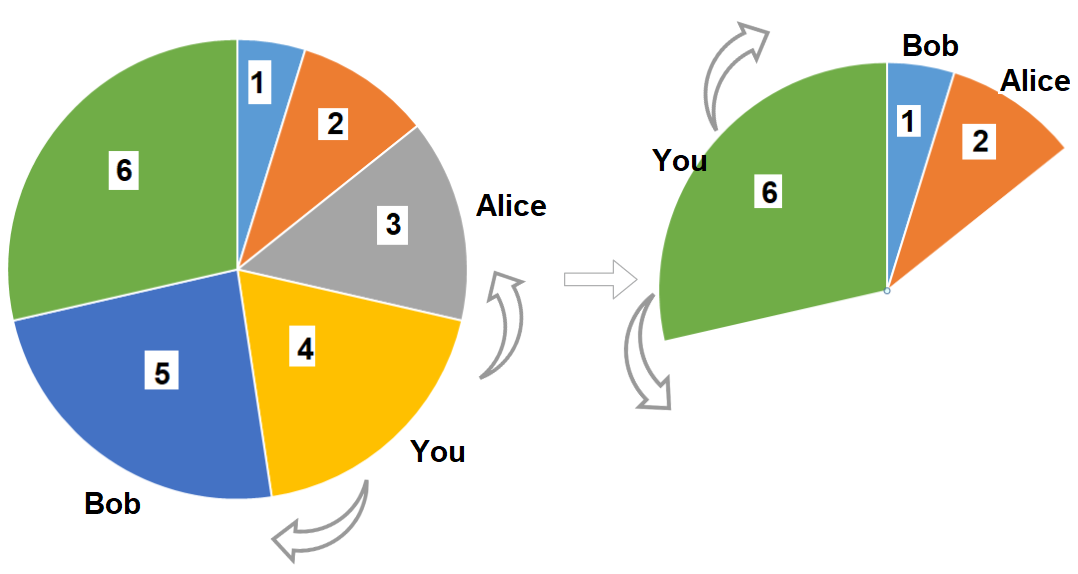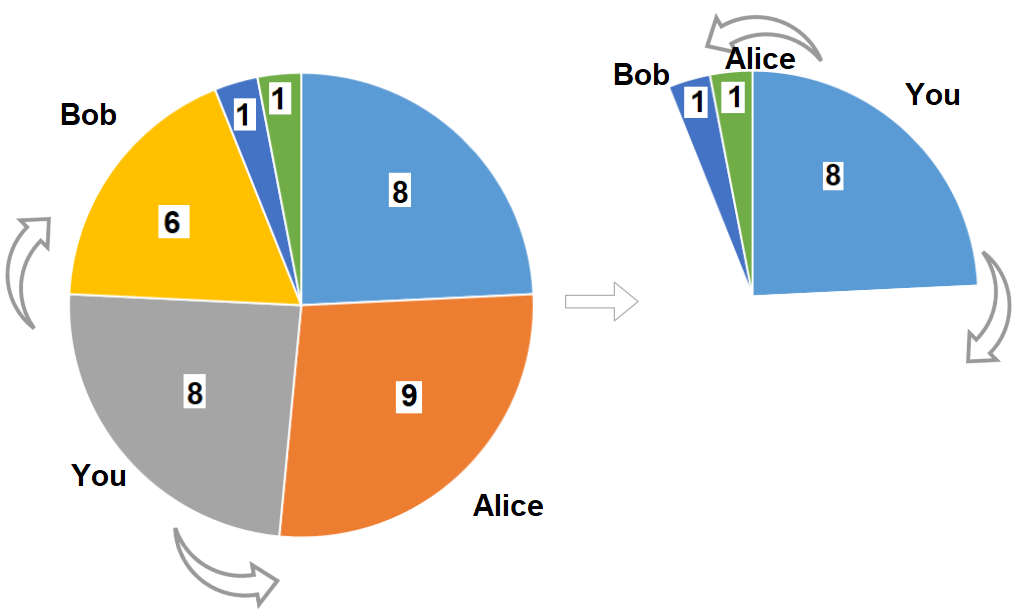Leetcode 1388：3n块披萨（超详细的解法！！！）
in leetcode with 0 comment

• 你挑选 任意 一块披萨。
• Alice 将会挑选你所选择的披萨逆时针方向的下一块披萨。
• Bob 将会挑选你所选择的披萨顺时针方向的下一块披萨。
• 重复上述过程直到没有披萨剩下。输入：slices = [1,2,3,4,5,6]输入：slices = [8,9,8,6,1,1]

输入：slices = [4,1,2,5,8,3,1,9,7]

输入：slices = [3,1,2]

• 1 <= slices.length <= 500
• slices.length % 3 == 0
• 1 <= slices[i] <= 1000

from functools import lru_cache
class Solution:
def maxSizeSlices(self, nums: List[int]) -> int:
n = len(nums)

@lru_cache(None)
def dfs(cur, r, k):
if k == 0: return 0
if cur >= r: return 0
return max(dfs(cur + 1, r, k), dfs(cur + 2, r, k - 1) + nums[cur])
return max(dfs(0, n - 1, n // 3), dfs(1, n, n // 3))

class Solution:
def maxSizeSlices(self, nums: List[int]) -> int:
n = len(nums)
k = n // 3

def cal(l, r):
dp = [ * (k + 1) for _ in range(n + 2)]
for i in range(l, r):
for j in range(k):
dp[i][j] = max(dp[i - 1][j], dp[i - 2][j - 1] + nums[i])
return dp[r - 1][k - 1]
return max(cal(0, n - 1), cal(1, n))

class Solution:
def maxSizeSlices(self, nums: List[int]) -> int:
n = len(nums)
k = n // 3

def cal(l, r):
dp = [ * (k + 1) for _ in range(2)]
for i in range(l, r):

dp, dp = dp, [j and max(nums[i] + dp[j - 1], dp[j]) for j in range(k + 1)]
return dp[-1][-1]
return max(cal(0, n - 1), cal(1, n))

dp, dp = dp, [max(nums[i] + dp[j - 1], dp[j]) for j in range(k)] + 

class Solution:
def maxSizeSlices(self, nums: List[int]) -> int:
n = len(nums)
k = n // 3

def cal(l, r):
dp = [ * (k + 1) for _ in range(2)]
for i in range(l, r):
for j in range(k, 0, -1):
dp[j] = dp[j]
dp[j] = max(dp[j], dp[j - 1] + nums[i])
return dp[k]
return max(cal(0, n - 1), cal(1, n))

「如果我的文章对你有很大帮助，那么不妨～！」Responses